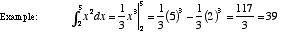index: click on a letter A B C D E F G H I J K L M N O P Q R S T U V W X Y Z A to Z index index: subject areas numbers & symbols sets, logic, proofs geometry algebra trigonometry advanced algebra & pre-calculus calculus advanced topics probability & statistics real world applications multimedia entrieswww.mathwords.com about mathwords website feedback

 Definite Integral An integral which is evaluated over an interval. A definite integral is written. Definite integrals are used to find the area between the graph of a function and the x-axis. There are many other applications. Formally, a definite integral is the limit of a Riemann sum as the norm of the partition approaches zero. That is,.See also